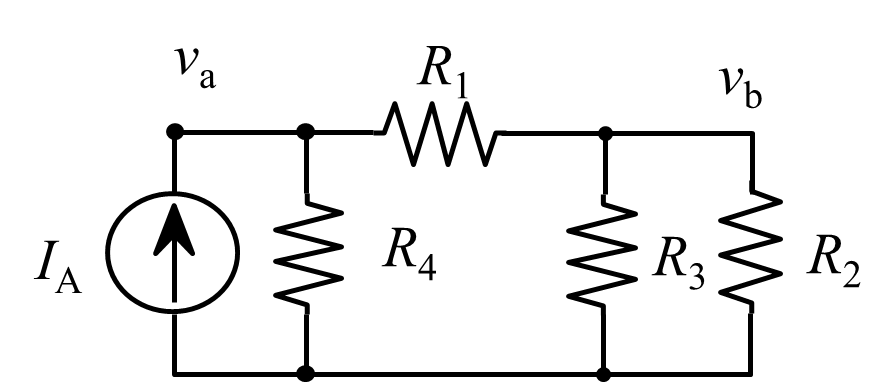# ECE 538 Assignment 5

1. Write a Java application that draws a resistor on the screen and uses three buttons to move the resistor up or down and rotate the resistor by 45-degrees. Use your most recent Resistor class.

2. Modify your resistor drawing program from the previous assignment by adding a JLabel to the bottom of the JFrame. Depending on the location of the mouse, the JLabel should display the name of the resistor and location from the center of the resistor if the mouse is over the resistor. See `MouseTracker.java` for some ideas.

3. Write a Java program to read a resistor net description from a file. Each line of the file should describe one resistor (space-delimited tokens) in the form `R name node1 node2 value x y angle`. where name is a string, node1 and node2 are integers, and the remaining variables are doubles. Your program should run even if some parameters (such as `x y angle`) are missing.

Store the resistor data in an `ArrayList` and print the data to a `TextArea`. Demonstrate using the netlist file circuit1.txt.

You should read the netlist from a file specified by `JFileChooser` (which should open in the current directory).

4. Modify the previous program so that it uses `Jama` to construct and solve the nodal analysis matrix equation A v = b for the nodal voltages v. Print the matrices and solution vector to a `TextArea`. Demonstrate using the netlist file circuit1.txt. Assume a current source of 1 mA flowing into node 1 from node 0 (ground) as shown in the circuit drawing below.5. Create a WIRE object that defines the connection (path) between two components (such as resistors). Read wire objects from your netlist file. A suitable example would be
```WIRE x1 y1 x2 y2 x3 y3
```

Extend your resistor drawing probram to read a netlist file and draw the corresponding circuit with wires.

Use your code to draw the circuit above (except for the current source)

6. Modify an image display program (such as LoadImageApp) to add a JLabel in BORDER.SOUTH that displays the location of the mouse and the color of the pixel at that location. Use a `JFileChooser` dialog to allow the user to select an image file.

Maintained by John Loomis, last updated 20 February 2019